# A spherical balloon is inflated so that its volume is increasing at the rate of 2 ft3/min

A spherical balloon is inflated so that its volume is increasing at the rate of 2 ft3/min How rapidly is the diameter of the balloon increasing when the diameter is 1.3 feet?

The diameter is increasing at _______ ft/min

SOLUTION :

Volume of ballon, V = 4/3 π r^3  = 4/3 π (d/2)^3

=> V = π / 6 * d^3

Differentiating w.r.t. t :

=> dV/dt = π/6 * 3 * d^2 d/dt (d) = π/2 * d^2 d/dt (d)

dV/dt at  d = 1.3 ft. Is 2 ft^3 / min

=> 2 = π /2 * (1.3)^2 *  d/dt (d)

=> 2 =  2.6546 d/dt (d)

=> d/dt (d) = 2 / 2.6546 = 0.7534  ft / min

So, diameter is increasing at the rate of 0.7534 ft / min when diameter is 1.3 ft . (ANSWER).

#### Earn Coin

Coins can be redeemed for fabulous gifts.

Similar Homework Help Questions
• ### A spherical balloon is inflated so that its volume is increasing at the rate of 3 ft 3/min. How fast is the radius of the balloon increasing when the radius is 1 ft?

A spherical balloon is inflated so that its volume is increasing at the rate of 3 ft 3/min. How fast is the radius of the balloon increasing when the radius is 1 ft?

• ### A spherical balloon is being inflated so that its volume is increasing at the rate of 200 cm3/min

A spherical balloon is being inflated so that its volume is increasing at the rate of 200 cm3/min. At what rate is the radius increasing when the radius is 15 cm.

• ### 3.9 1. Balloons A spherical balloon is inflated and its volume increases at a rate of...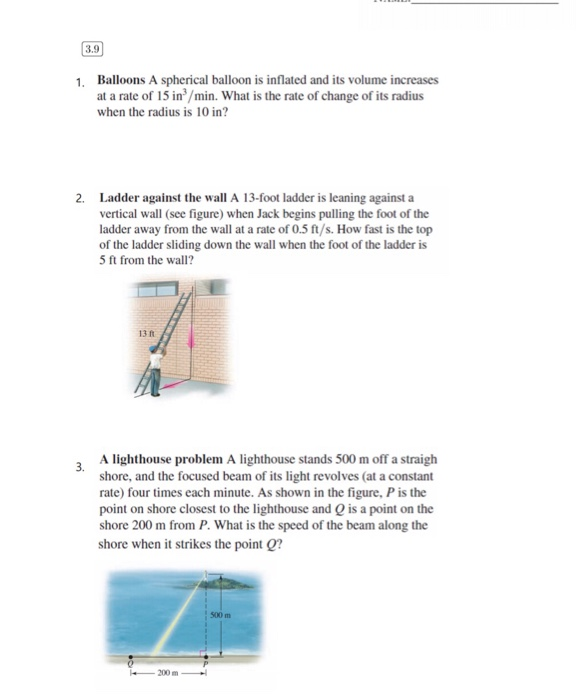3.9 1. Balloons A spherical balloon is inflated and its volume increases at a rate of 15 in/min. What is the rate of change of its radius when the radius is 10 in? 2. Ladder against the wall A 13-foot ladder is leaning against a vertical wall (see figure) when Jack begins pulling the foot of the ladder away from the wall at a rate of 0.5 ft/s. How fast is the top of the ladder sliding down the wall...

• ### 4. A spherical balloon is inflated at a rate of 10 cubic feet per minute. How...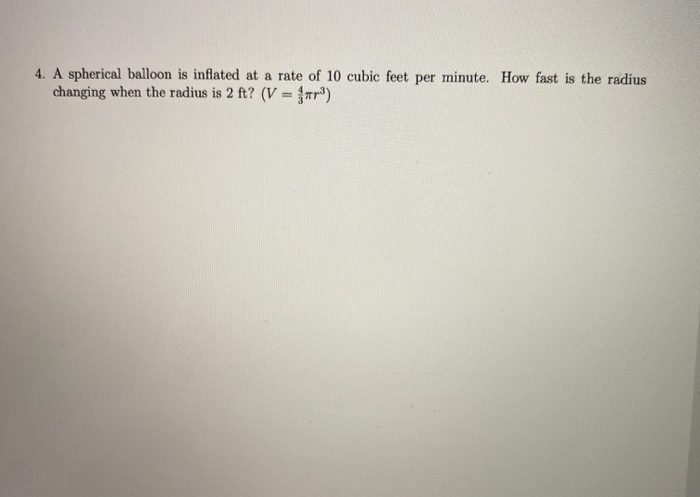4. A spherical balloon is inflated at a rate of 10 cubic feet per minute. How fast is the radius changing when the radius is 2 ft? (V = far)

• ### A spherical party balloon is being inflated with helium pumped in at a rate of 5...continues

A spherical party balloon is being inflated with helium pumped in at a rate of 5 cubic feet per minute. How fast is the radius growing at the instant when the radiushas reached 1 ft? HINT [See Example 1.] (The volume of a sphere of radius r is V = 4/3?r3. Round your answer to two decimal places.)

• ### 6. (4 pts) Air is being pumped into a spherical balloon so that its volume increases...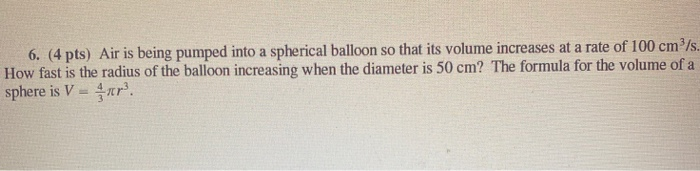6. (4 pts) Air is being pumped into a spherical balloon so that its volume increases at a rate of 100 cm?/s. How fast is the radius of the balloon increasing when the diameter is 50 cm? The formula for the volume of a sphere is V = r.

• ### A spherical balloon is inflating with helium at a rate of 641 it? min How fast...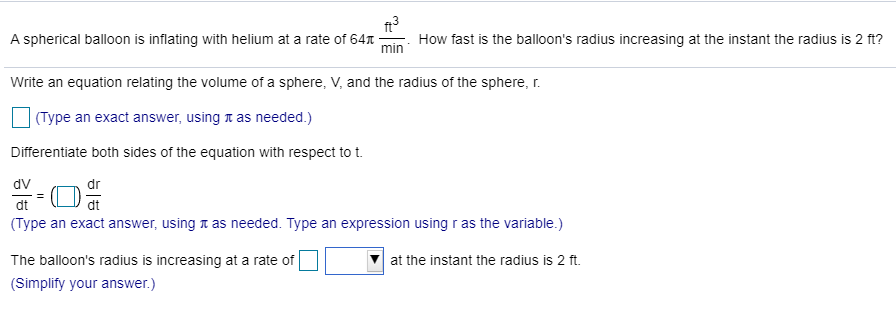A spherical balloon is inflating with helium at a rate of 641 it? min How fast is the balloon's radius increasing at the instant the radius is 2 ft? Write an equation relating the volume of a sphere, V, and the radius of the sphere, r. (Type an exact answer, using a as needed.) Differentiate both sides of the equation with respect to t. dv dr dt (Type an exact answer, using a as needed. Type an expression using r...

• ### A spherical balloon is inflated with gas at a rate of 900 cubic centimeters per minute....

A spherical balloon is inflated with gas at a rate of 900 cubic centimeters per minute. (a) Find the rates of change of the radius when r = 40 centimeters and r = 75 centimeters. r = 40 cm/min r = 75 cm/min (b) Explain why the rate of change of the radius of the sphere is not constant even though dV/dt is constant. The rate of change of the radius is a linear relationship whose slope is dV dt...

• ### Air is blown into a spherical balloon so that, when its radius is 6.60 cm, its...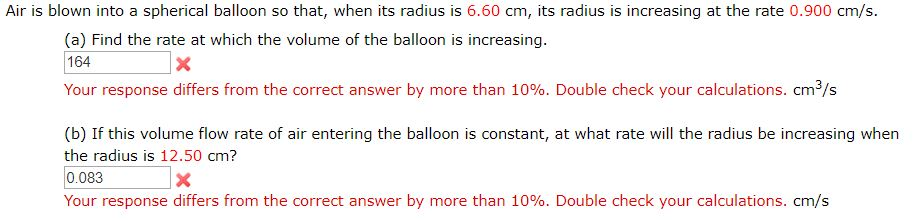Air is blown into a spherical balloon so that, when its radius is 6.60 cm, its radius is increasing at the rate 0.900 cm/s. (a) Find the rate at which the volume of the balloon is increasing. 164 Your response differs from the correct answer by more than 10%. Double check your calculations. cm/s (b) If this volume flow rate of air entering the balloon is constant, at what rate will the radius be increasing when the radius is 12.50...

• ### RATES OF CHANGE QUESTION A spherical balloon is being blown up so that its volume is increasing by 0.6 m^3 s^-1

RATES OF CHANGE QUESTIONA spherical balloon is being blown up so that its volume is increasing by 0.6 m^3 s^-1. Find the rate at which the radius is increasing when the radius is 0.1 m.So what I did was that I determined dV/dt =0.6and dV/dr = 4/3 r^3 phi (which is the volume of a sphere), and I should calculate dr/dt.The answer should be 15 / phi but I figured I'll have to inverse the dV/dr part in order to...